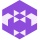Home Communities
IT Knowledge
Inspiration
Languages
EN

# Python - math.sqrt() method example

0 points
Created by:Dale-K1
327

`math.sqrt` is a method that returns a number which is the square root of input value. The method works only on positive real numbers.

``````import math

print( math.sqrt(  4   ) ) # 2
print( math.sqrt(  9   ) ) # 3

print( math.sqrt(  2   ) ) # 1.4142135623730951
print( math.sqrt(  0.5 ) ) # 0.7071067811865476
print( math.sqrt(  0   ) ) # 0
print( math.sqrt( -1   ) ) # ValueError``````

## 1. Documentation

 Syntax ``math.sqrt(x)`` Parameters `x` - number to find the square of. Value in the range `0` to `infinity`. Result Square root `number` value in range `0` to `infinity`. If the operation can not be executed `ValueError` is returned. Description `sqrt` is a method that returns a number which is square root of input value. The method works only on positive real numbers.

## 2. Square root with `math.pow` method examples

In this example, the way how to calculate square root using power function is presented.

``````import math

def calculate_sqrt(value):
return math.pow(value, 0.5)

#Examples:
print( calculate_sqrt(  4   ) ) # 2
print( calculate_sqrt(  9   ) ) # 3

print( calculate_sqrt(  2   ) ) # 1.4142135623730951
print( calculate_sqrt(  0.5 ) ) # 0.7071067811865476
print( calculate_sqrt(  0   ) ) # 0
print( calculate_sqrt( -1   ) ) # ValueError``````
Join to our subscribers to be up to date with content, news and offers.